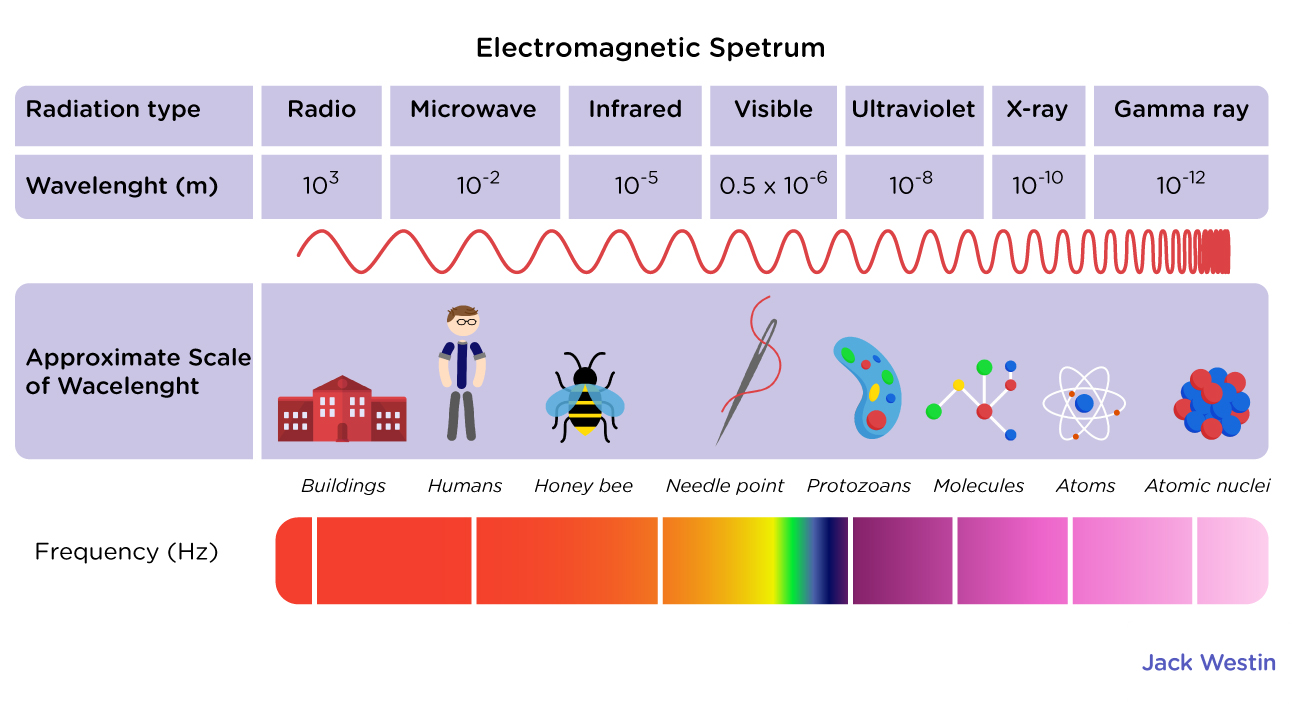Having trouble writing your personal statement?

MCAT Content / Light And Electromagnetic Radiation / Classification Of Electromagnetic Spectrum Photon Energy E Hf

### Classification of electromagnetic spectrum, photon energy E = hf

The electromagnetic spectrum is a wide collection of different waves with varying frequencies, wavelengths and properties.

The lowest frequency portion of the electromagnetic spectrum is designated as “radio,” generally considered to have wavelengths within 1 millimeter to 100 kilometers or frequencies within 300 GHz to 3 kHz. The corresponding wavelength is the longest from 1 millimeter to 100 kilometers. Radio waves are generally used for telecommunications.

On the other end of the spectrum, Gamma rays are the highest energy EM radiation and typically have energies greater than 100 keV, frequencies greater than 10^19 Hz, and wavelengths less than 10 picometers.

Visible light is the portion of the electromagnetic spectrum that is visible to the human eye. Electromagnetic radiation in this range of wavelengths is often simply referred to as “light”. A typical human eye will respond to wavelengths from about 390 to 750 nm (0.39 to 0.75 µm). In terms of frequency, this corresponds to a band in the vicinity of 400–790 THz.Photon energy can be calculated by using the frequency of the light particles emitted from an object and multiplying by Planck’s constant to work out the energy.

E = hf

Where h = 6.62607015×10−34 J⋅s. is Planck constant. This states that the energy of a photon depends directly on its frequency. By substituting wave properties, this equation can also be written as E = hc/λ.

Practice Questions

MCAT Official Prep

Official Guide C/P Section Passage 2 Question 6

Physics Question Pack Passage 18 Question 107

Key Points

• The electromagnetic spectrum goes from low to high frequency: Radiowaves – Microwaves – Infrared – Visible Light – UltraViolet – X-Rays – Gamma Rays.

Key Terms

Planck constant: h = 6.62607015×10−34 J⋅s

photon: a type of elementary particle such as light and radio waves

Billing Information